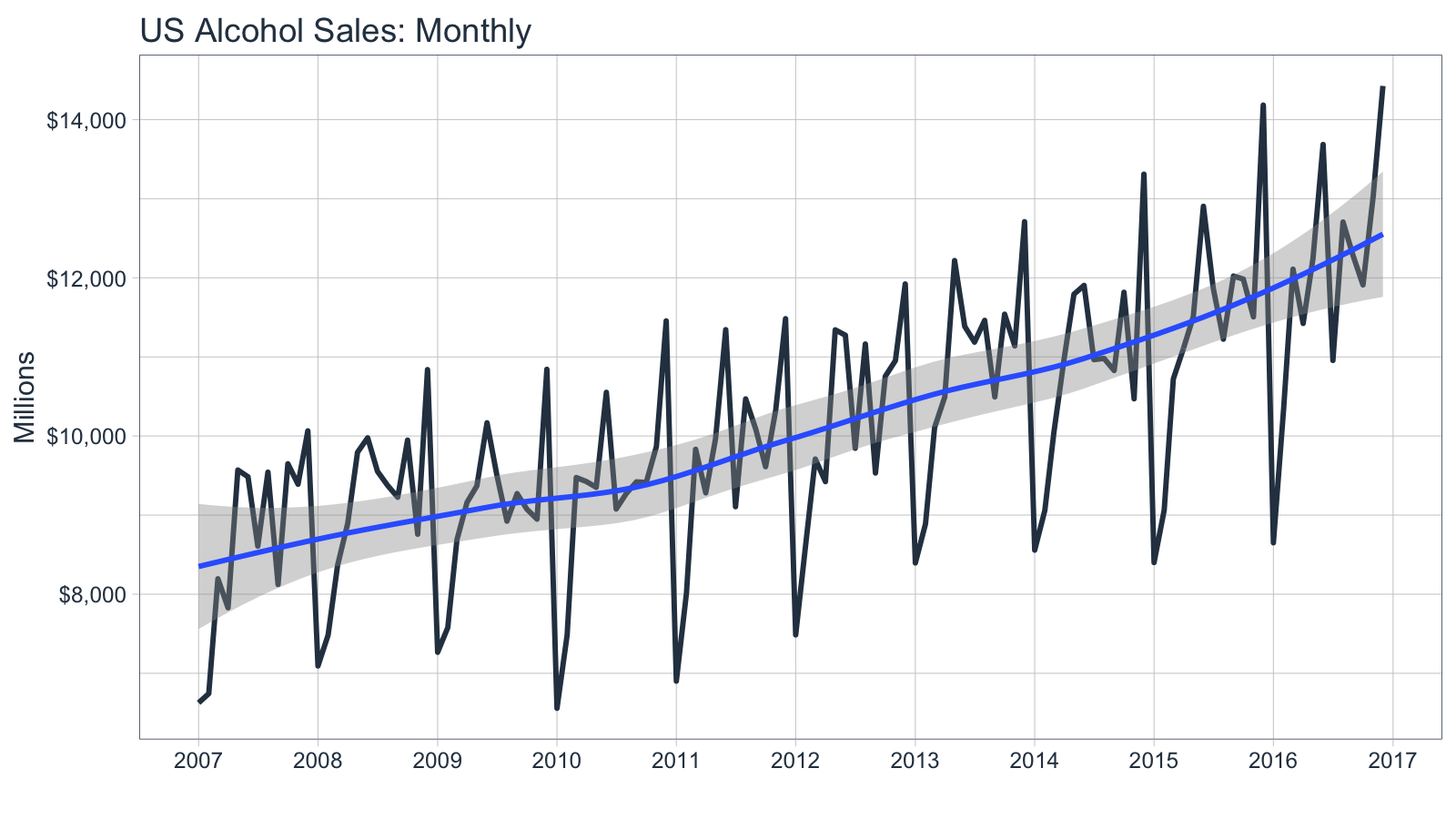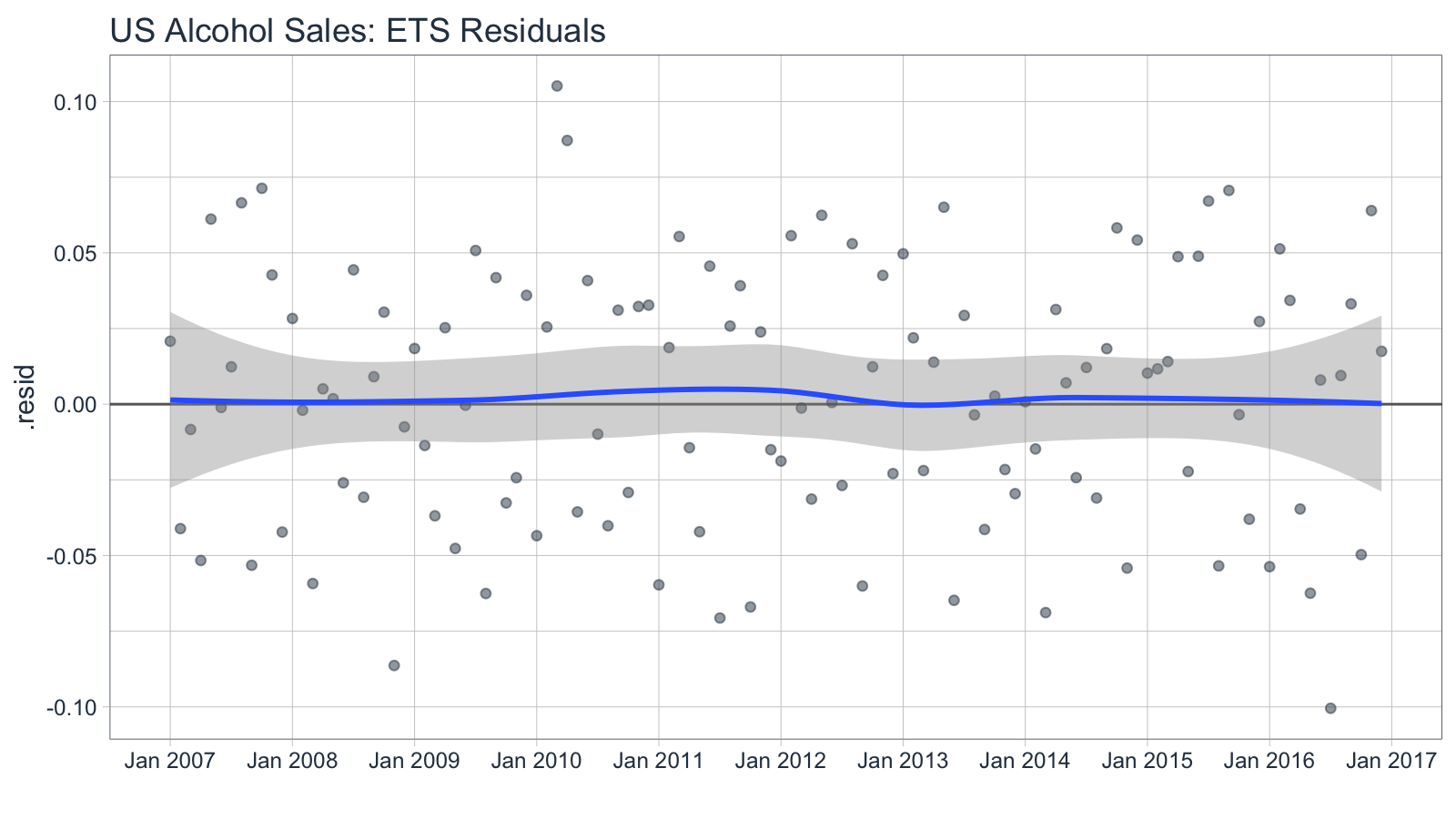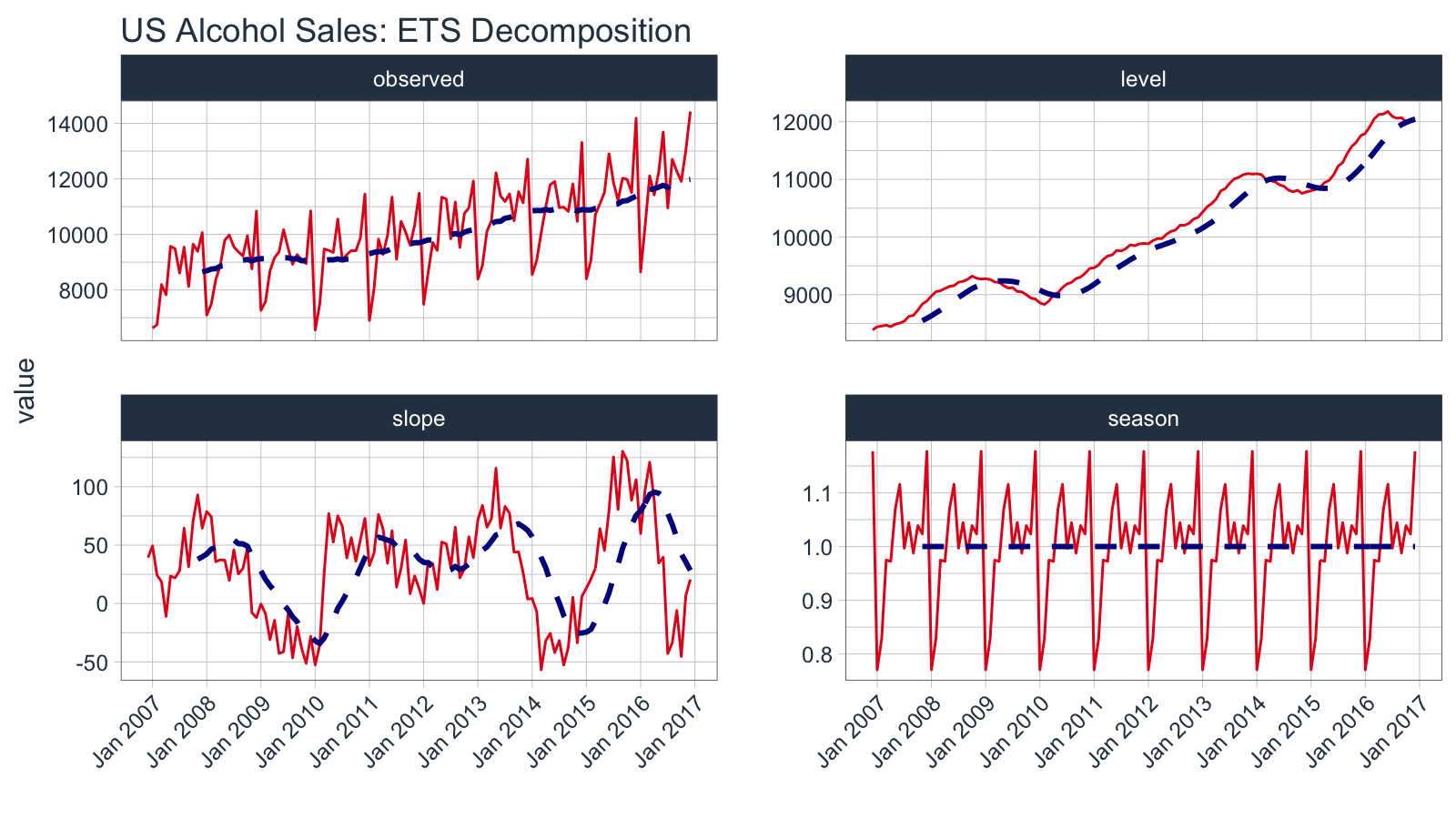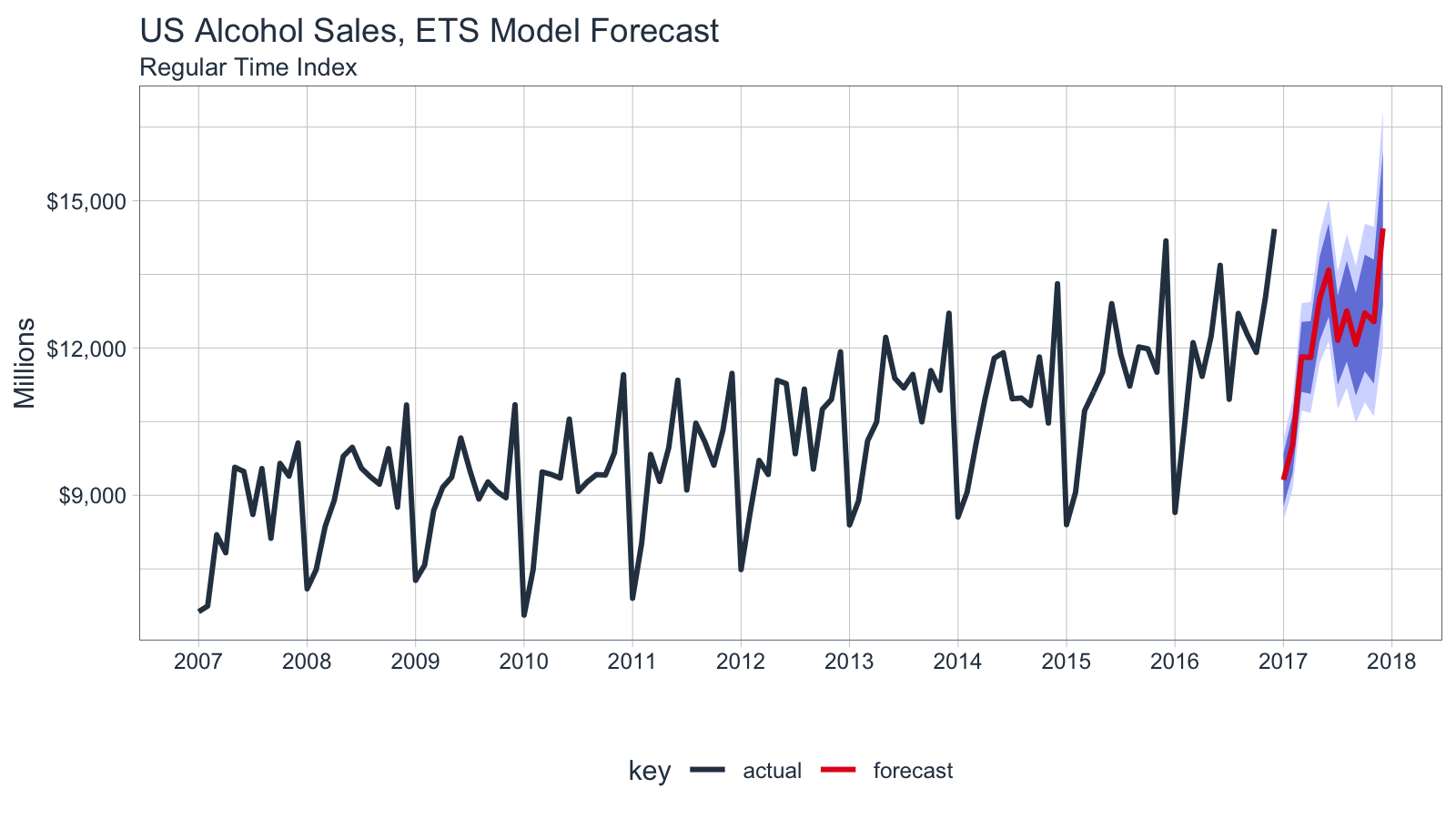# Introduction to sweep

#### 2020-07-10

Extending `broom` to time series forecasting

The `sweep` package extends the `broom` tools (tidy, glance, and augment) for performing forecasts and time series analysis in the “tidyverse”. The package is geared towards the workflow required to perform forecasts using Rob Hyndman’s `forecast` package, and contains the following elements:

1. model tidiers: `sw_tidy`, `sw_glance`, `sw_augment`, `sw_tidy_decomp` functions extend `tidy`, `glance`, and `augment` from the `broom` package specifically for models (`ets()`, `Arima()`, `bats()`, etc) used for forecasting.

2. forecast tidier: `sw_sweep` converts a `forecast` object to a tibble that can be easily manipulated in the “tidyverse”.

To illustrate, let’s take a basic forecasting workflow starting from data collected in a tibble format and then performing a forecast to achieve the end result in tibble format.

# Prerequisites

Before we get started, load the following packages.

``library(tidyverse)``
``## Warning: package 'ggplot2' was built under R version 3.6.2``
``## Warning: package 'tidyr' was built under R version 3.6.2``
``## Warning: package 'purrr' was built under R version 3.6.2``
``library(tidyquant)``
``## Warning: package 'lubridate' was built under R version 3.6.2``
``## Warning: package 'zoo' was built under R version 3.6.2``
``````library(timetk)
library(sweep)
library(forecast)``````
``## Warning: package 'forecast' was built under R version 3.6.2``

# Forecasting Sales of Beer, Wine, and Distilled Alcohol Beverages

We’ll use the `tidyquant` package to get the US alcohol sales, which comes from the FRED data base (the origin is the US Bureau of the Census, one of the 80+ data sources FRED connects to). The FRED code is “S4248SM144NCEN” and the data set can be found here.

``````alcohol_sales_tbl <- tq_get("S4248SM144NCEN",
get  = "economic.data",
from = "2007-01-01",
to   = "2016-12-31")
alcohol_sales_tbl``````
``````## # A tibble: 120 x 3
##    symbol         date       price
##    <chr>          <date>     <int>
##  1 S4248SM144NCEN 2007-01-01  6627
##  2 S4248SM144NCEN 2007-02-01  6743
##  3 S4248SM144NCEN 2007-03-01  8195
##  4 S4248SM144NCEN 2007-04-01  7828
##  5 S4248SM144NCEN 2007-05-01  9570
##  6 S4248SM144NCEN 2007-06-01  9484
##  7 S4248SM144NCEN 2007-07-01  8608
##  8 S4248SM144NCEN 2007-08-01  9543
##  9 S4248SM144NCEN 2007-09-01  8123
## 10 S4248SM144NCEN 2007-10-01  9649
## # … with 110 more rows``````

We can quickly visualize using the `ggplot2` package. We can see that there appears to be some seasonality and an upward trend.

``````alcohol_sales_tbl %>%
ggplot(aes(x = date, y = price)) +
geom_line(size = 1, color = palette_light()[]) +
geom_smooth(method = "loess") +
labs(title = "US Alcohol Sales: Monthly", x = "", y = "Millions") +
scale_y_continuous(labels = scales::dollar) +
scale_x_date(date_breaks = "1 year", date_labels = "%Y") +
theme_tq()``````
``## `geom_smooth()` using formula 'y ~ x'``# Forecasting Workflow

The forecasting workflow involves a few basic steps:

1. Step 1: Coerce to a `ts` object class.
2. Step 2: Apply a model (or set of models)
3. Step 3: Forecast the models (similar to predict)
4. Step 4: Use `sw_sweep()` to tidy the forecast.

Note that we purposely omit other steps such as testing the series for stationarity (`Box.test(type = "Ljung")`) and analysis of autocorrelations (`Acf`, `Pacf`) for brevity purposes. We recommend the analyst to follow the forecasting workflow in “Forecasting: principles and practice”

## Step 1: Coerce to a `ts` object class

The `forecast` package uses the `ts` data structure, which is quite a bit different than tibbles that we are currently using. Fortunately, it’s easy to get to the correct structure with `tk_ts()` from the `timetk` package. The `start` and `freq` variables are required for the regularized time series (`ts`) class, and these specify how to treat the time series. For monthly, the frequency should be specified as 12. This results in a nice calendar view. The `silent = TRUE` tells the `tk_ts()` function to skip the warning notifying us that the “date” column is being dropped. Non-numeric columns must be dropped for `ts` class, which is matrix based and a homogeneous data class.

``````alcohol_sales_ts <- tk_ts(alcohol_sales_tbl, start = 2007, freq = 12, silent = TRUE)
alcohol_sales_ts``````
``````##        Jan   Feb   Mar   Apr   May   Jun   Jul   Aug   Sep   Oct   Nov   Dec
## 2007  6627  6743  8195  7828  9570  9484  8608  9543  8123  9649  9390 10065
## 2008  7093  7483  8365  8895  9794  9977  9553  9375  9225  9948  8758 10839
## 2009  7266  7578  8688  9162  9369 10167  9507  8923  9272  9075  8949 10843
## 2010  6558  7481  9475  9424  9351 10552  9077  9273  9420  9413  9866 11455
## 2011  6901  8014  9832  9281  9967 11344  9106 10469 10085  9612 10328 11483
## 2012  7486  8641  9709  9423 11342 11274  9845 11163  9532 10754 10953 11922
## 2013  8395  8888 10110 10493 12218 11385 11186 11462 10494 11540 11138 12709
## 2014  8557  9059 10055 10977 11792 11904 10965 10981 10828 11817 10470 13310
## 2015  8400  9062 10722 11107 11508 12904 11869 11224 12022 11983 11506 14183
## 2016  8650 10324 12109 11423 12242 13685 10955 12705 12276 11910 13021 14425``````

A significant benefit is that the resulting `ts` object maintains a “timetk index”, which will help with forecasting dates later. We can verify this using `has_timetk_idx()` from the `timetk` package.

``has_timetk_idx(alcohol_sales_ts)``
``##  TRUE``

Now that a time series has been coerced, let’s proceed with modeling.

## Step 2: Modeling a time series

The modeling workflow takes a time series object and applies a model. Nothing new here: we’ll simply use the `ets()` function from the `forecast` package to get an Exponential Smoothing ETS (Error, Trend, Seasonal) model.

``````fit_ets <- alcohol_sales_ts %>%
ets()``````

Where `sweep` can help is in the evaluation of a model. Expanding on the `broom` package there are four functions:

• `sw_tidy()`: Returns a tibble of model parameters
• `sw_glance()`: Returns the model accuracy measurements
• `sw_augment()`: Returns the fitted and residuals of the model
• `sw_tidy_decomp()`: Returns a tidy decomposition from a model

The guide below shows which model object compatibility with `sweep` tidier functions.

Function Compatibility
Object sw_tidy() sw_glance() sw_augment() sw_tidy_decomp() sw_sweep()
ar
arima X X X
Arima X X X
ets X X X X
baggedETS
bats X X X X
tbats X X X X
nnetar X X X
stl X
HoltWinters X X X X
StructTS X X X X
tslm X X X
decompose X
Box.test X X
kpss.test X X
forecast X

Going through the tidiers, we can get useful `model` information.

### sw_tidy

`sw_tidy()` returns the model parameters.

``sw_tidy(fit_ets)``
``````## # A tibble: 17 x 2
##    term     estimate
##    <chr>       <dbl>
##  1 alpha    0.101
##  2 beta     0.0659
##  3 gamma    0.000436
##  4 phi      0.961
##  5 l     8387.
##  6 b       39.2
##  7 s0       1.18
##  8 s1       1.02
##  9 s2       1.04
## 10 s3       0.988
## 11 s4       1.04
## 12 s5       0.997
## 13 s6       1.12
## 14 s7       1.07
## 15 s8       0.972
## 16 s9       0.975
## 17 s10      0.828``````

### sw_glance

`sw_glance()` returns the model quality parameters.

``sw_glance(fit_ets)``
``````## # A tibble: 1 x 12
##   model.desc  sigma logLik   AIC   BIC    ME  RMSE   MAE    MPE  MAPE  MASE
##   <chr>       <dbl>  <dbl> <dbl> <dbl> <dbl> <dbl> <dbl>  <dbl> <dbl> <dbl>
## 1 ETS(M,Ad,… 0.0454 -1012. 2059. 2110.  15.2  430.  358. 0.0130  3.54 0.691
## # … with 1 more variable: ACF1 <dbl>``````

### sw_augment

`sw_augment()` returns the actual, fitted and residual values.

``````augment_fit_ets <- sw_augment(fit_ets)
augment_fit_ets``````
``````## # A tibble: 120 x 4
##    index     .actual .fitted   .resid
##    <yearmon>   <dbl>   <dbl>    <dbl>
##  1 Jan 2007     6627   6492.  0.0208
##  2 Feb 2007     6743   7032. -0.0411
##  3 Mar 2007     8195   8264. -0.00835
##  4 Apr 2007     7828   8254. -0.0516
##  5 May 2007     9570   9018.  0.0612
##  6 Jun 2007     9484   9494. -0.00108
##  7 Jul 2007     8608   8503.  0.0124
##  8 Aug 2007     9543   8948.  0.0665
##  9 Sep 2007     8123   8580. -0.0532
## 10 Oct 2007     9649   9007.  0.0713
## # … with 110 more rows``````

We can review the residuals to determine if their are any underlying patterns left. Note that the index is class `yearmon`, which is a regularized date format.

``````augment_fit_ets %>%
ggplot(aes(x = index, y = .resid)) +
geom_hline(yintercept = 0, color = "grey40") +
geom_point(color = palette_light()[], alpha = 0.5) +
geom_smooth(method = "loess") +
scale_x_yearmon(n = 10) +
labs(title = "US Alcohol Sales: ETS Residuals", x = "") +
theme_tq()``````
``## `geom_smooth()` using formula 'y ~ x'``### sw_tidy_decomp

`sw_tidy_decomp()` returns the decomposition of the ETS model.

``````decomp_fit_ets <- sw_tidy_decomp(fit_ets)
decomp_fit_ets ``````
``````## # A tibble: 121 x 5
##    index     observed level slope season
##    <yearmon>    <dbl> <dbl> <dbl>  <dbl>
##  1 Dec 2006        NA 8387.  39.2  1.18
##  2 Jan 2007      6627 8442.  49.3  0.771
##  3 Feb 2007      6743 8454.  24.3  0.828
##  4 Mar 2007      8195 8471.  18.7  0.975
##  5 Apr 2007      7828 8444. -11.0  0.972
##  6 May 2007      9570 8486.  23.5  1.07
##  7 Jun 2007      9484 8508.  21.9  1.12
##  8 Jul 2007      8608 8539.  28.0  0.997
##  9 Aug 2007      9543 8624.  64.5  1.04
## 10 Sep 2007      8123 8639.  31.5  0.988
## # … with 111 more rows``````

We can review the decomposition using `ggplot2` as well. The data will need to be manipulated slightly for the facet visualization. The `gather()` function from the `tidyr` package is used to reshape the data into a long format data frame with column names “key” and “value” indicating all columns except for index are to be reshaped. The “key” column is then mutated using `mutate()` to a factor which preserves the order of the keys so “observed” comes first when plotting.

``````decomp_fit_ets %>%
gather(key = key, value = value, -index) %>%
mutate(key = forcats::as_factor(key)) %>%
ggplot(aes(x = index, y = value, group = key)) +
geom_line(color = palette_light()[]) +
geom_ma(ma_fun = SMA, n = 12, size = 1) +
facet_wrap(~ key, scales = "free_y") +
scale_x_yearmon(n = 10) +
labs(title = "US Alcohol Sales: ETS Decomposition", x = "") +
theme_tq() +
theme(axis.text.x = element_text(angle = 45, hjust = 1))``````
``## Warning: Removed 1 row(s) containing missing values (geom_path).``Under normal circumstances it would make sense to refine the model at this point. However, in the interest of showing capabilities (rather than how to forecast) we move onto forecasting the model. For more information on how to forecast, please refer to the online book “Forecasting: principles and practices”.

## Step 3: Forecasting the model

Next we forecast the ETS model using the `forecast()` function. The returned `forecast` object isn’t in a “tidy” format (i.e. data frame). This is where the `sw_sweep()` function helps.

``````fcast_ets <- fit_ets %>%
forecast(h = 12) ``````

## Step 4: Tidy the forecast object

We’ll use the `sw_sweep()` function to coerce a `forecast` into a “tidy” data frame. The `sw_sweep()` function then coerces the `forecast` object into a tibble that can be sent to `ggplot` for visualization. Let’s inspect the result.

``sw_sweep(fcast_ets, fitted = TRUE)``
``````## # A tibble: 252 x 7
##    index     key    price lo.80 lo.95 hi.80 hi.95
##    <yearmon> <chr>  <dbl> <dbl> <dbl> <dbl> <dbl>
##  1 Jan 2007  actual  6627    NA    NA    NA    NA
##  2 Feb 2007  actual  6743    NA    NA    NA    NA
##  3 Mar 2007  actual  8195    NA    NA    NA    NA
##  4 Apr 2007  actual  7828    NA    NA    NA    NA
##  5 May 2007  actual  9570    NA    NA    NA    NA
##  6 Jun 2007  actual  9484    NA    NA    NA    NA
##  7 Jul 2007  actual  8608    NA    NA    NA    NA
##  8 Aug 2007  actual  9543    NA    NA    NA    NA
##  9 Sep 2007  actual  8123    NA    NA    NA    NA
## 10 Oct 2007  actual  9649    NA    NA    NA    NA
## # … with 242 more rows``````

The tibble returned contains “index”, “key” and “value” (or in this case “price”) columns in a long or “tidy” format that is ideal for visualization with `ggplot2`. The “index” is in a regularized format (in this case `yearmon`) because the `forecast` package uses `ts` objects. We’ll see how we can get back to the original irregularized format (in this case `date`) later. The “key” and “price” columns contains three groups of key-value pairs:

1. actual: the actual values from the original data
2. fitted: the model values returned from the `ets()` function (excluded by default)
3. forecast: the predicted values from the `forecast()` function

The `sw_sweep()` function contains an argument `fitted = FALSE` by default meaning that the model “fitted” values are not returned. We can toggle this on if desired. The remaining columns are the forecast confidence intervals (typically 80 and 95, but this can be changed with `forecast(level = c(80, 95))`). These columns are setup in a wide format to enable using the `geom_ribbon()`.

Let’s visualize the forecast with `ggplot2`. We’ll use a combination of `geom_line()` and `geom_ribbon()`. The fitted values are toggled off by default to reduce the complexity of the plot, but these can be added if desired. Note that because we are using a regular time index of the `yearmon` class, we need to add `scale_x_yearmon()`.

``````sw_sweep(fcast_ets) %>%
ggplot(aes(x = index, y = price, color = key)) +
geom_ribbon(aes(ymin = lo.95, ymax = hi.95),
fill = "#D5DBFF", color = NA, size = 0) +
geom_ribbon(aes(ymin = lo.80, ymax = hi.80, fill = key),
fill = "#596DD5", color = NA, size = 0, alpha = 0.8) +
geom_line(size = 1) +
labs(title = "US Alcohol Sales, ETS Model Forecast", x = "", y = "Millions",
subtitle = "Regular Time Index") +
scale_y_continuous(labels = scales::dollar) +
scale_x_yearmon(n = 12, format = "%Y") +
scale_color_tq() +
scale_fill_tq() +
theme_tq() ``````Because the `ts` object was created with the `tk_ts()` function, it contained a timetk index that was carried with it throughout the forecasting workflow. As a result, we can use the `timetk_idx` argument, which maps the original irregular index (dates) and a generated future index to the regularized time series (yearmon). This results in the ability to return an index of date and datetime, which is not currently possible with the `forecast` objects. Notice that the index is returned as `date` class.

``````sw_sweep(fcast_ets, timetk_idx = TRUE) %>%
``````## # A tibble: 6 x 7
##   index      key    price lo.80 lo.95 hi.80 hi.95
##   <date>     <chr>  <dbl> <dbl> <dbl> <dbl> <dbl>
## 1 2007-01-01 actual  6627    NA    NA    NA    NA
## 2 2007-02-01 actual  6743    NA    NA    NA    NA
## 3 2007-03-01 actual  8195    NA    NA    NA    NA
## 4 2007-04-01 actual  7828    NA    NA    NA    NA
## 5 2007-05-01 actual  9570    NA    NA    NA    NA
## 6 2007-06-01 actual  9484    NA    NA    NA    NA``````
``````sw_sweep(fcast_ets, timetk_idx = TRUE) %>%
tail()``````
``````## # A tibble: 6 x 7
##   index      key       price  lo.80  lo.95  hi.80  hi.95
##   <date>     <chr>     <dbl>  <dbl>  <dbl>  <dbl>  <dbl>
## 1 2017-07-01 forecast 12156. 11246. 10765. 13065. 13547.
## 2 2017-08-01 forecast 12751. 11726. 11183. 13777. 14320.
## 3 2017-09-01 forecast 12074. 11027. 10473. 13121. 13675.
## 4 2017-10-01 forecast 12713. 11525. 10895. 13902. 14531.
## 5 2017-11-01 forecast 12537. 11273. 10604. 13801. 14470.
## 6 2017-12-01 forecast 14437. 12871. 12041. 16004. 16833.``````

We can build the same plot with dates in the x-axis now.

``````sw_sweep(fcast_ets, timetk_idx = TRUE) %>%
ggplot(aes(x = index, y = price, color = key)) +
geom_ribbon(aes(ymin = lo.95, ymax = hi.95),
fill = "#D5DBFF", color = NA, size = 0) +
geom_ribbon(aes(ymin = lo.80, ymax = hi.80, fill = key),
fill = "#596DD5", color = NA, size = 0, alpha = 0.8) +
geom_line(size = 1) +
labs(title = "US Alcohol Sales, ETS Model Forecast", x = "", y = "Millions",
subtitle = "Irregular Time Index") +
scale_y_continuous(labels = scales::dollar) +
scale_x_date(date_breaks = "1 year", date_labels = "%Y") +
scale_color_tq() +
scale_fill_tq() +
theme_tq() ``````